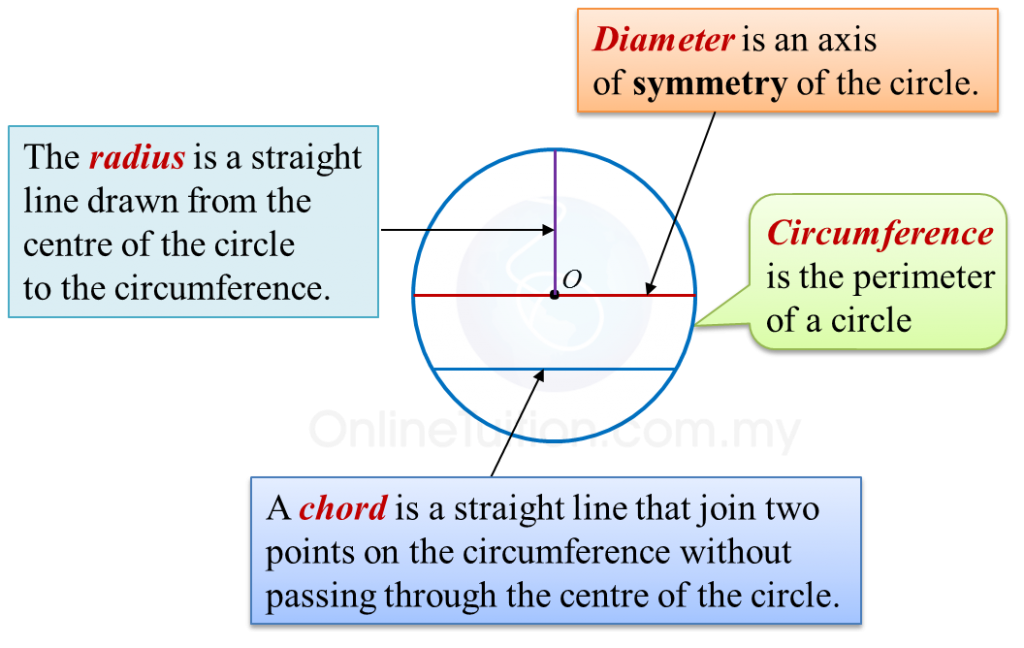10.1 Circles I

10.1 Circles I

10.1.1 Parts of a Circle
1. A circle is set of points in a plane equidistant from a fixed point.

2.
Parts of a circle:
(a)    The centre, O, of a circle is a fixed point which is equidistant from all points on the circle.(b)
A sector is the region enclosed by two radii and an arc.

(c)
An arc is a part of the circumference of a circle.

(d)
A segment is an area enclosed by an arc and a chord.

10.1.2 Circumference of a Circle

Example:
Calculate the circumference of a circle with a diameter of 14 cm. $\left(\pi =\frac{22}{7}\right)$

Solution
:
$\begin{array}{l}\text{Circumference}=\pi ×\text{Diameter}\\ \text{}=\frac{22}{7}×14\\ \text{}=44\text{cm}\end{array}$

10.1.3 Arc of a Circle
The length of an arc of a circle is proportional to the angle at the centre.

Example:

Calculate the length of the minor arc AB of the circle above. $\left(\pi =\frac{22}{7}\right)$

Solution
:
$\begin{array}{l}\frac{\text{Length of arc}}{\text{Circumference}}=\frac{\text{Angle at centre}}{{360}^{o}}\\ \\ \text{Length of arc}AB=\frac{{120}^{o}}{{360}^{o}}×2×\frac{22}{7}×7\\ \text{}=14\frac{2}{3}\text{cm}\end{array}$

10.1.4 Area of a Circle

Example:
Calculate the area of each of the following circles that has
(a) a radius of 7 cm,
(b)   a diameter of 10 cm.
$\left(\pi =\frac{22}{7}\right)$

Solution
:
(a)
$\begin{array}{l}\text{Area of a circle}=\pi {r}^{2}\\ \text{}=\frac{22}{\overline{)7}}×\overline{)7}×7\\ \text{}=154{\text{cm}}^{2}\end{array}$

(b)
$\begin{array}{l}\text{Diameter of circle}=10\text{cm}\\ \text{Radius of circle}=5\text{cm}\\ \text{Area of circle}=\pi {r}^{2}\\ \text{}=\frac{22}{7}×5×5\\ \text{}=78.57{\text{cm}}^{2}\end{array}$

10.1.5 Area of a Sector
The area of a sector of a circle is proportional to the angle at the centre.

Example
:

$\begin{array}{l}\text{Area of sector}ABC\\ =\frac{{72}^{o}}{{360}^{o}}×\frac{22}{7}×7×7\\ =30\frac{4}{5}{\text{cm}}^{2}\end{array}$